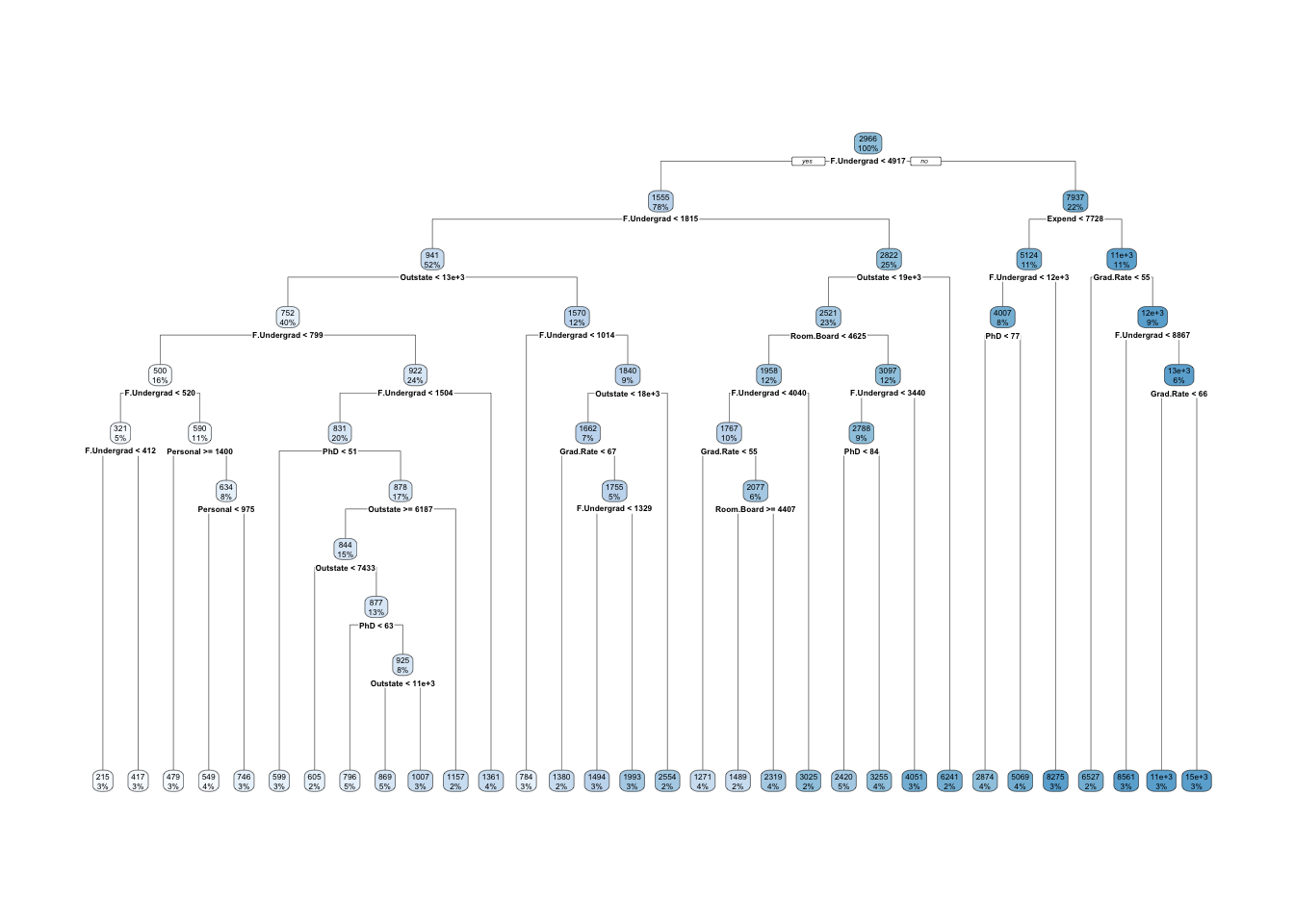library(tidymodels)
library(ISLR)
library(rpart.plot)

Using the College data from the ISLR package, predict the number of applications received from a subset of the variables of your choice using a decision tree. (Not sure about the variables? Run ?College in the console after loading the ISLR package)

I am going to first split the data set into a training and testing set. The training set will have 389 observations, the testing 388.

college_split <- initial_split(College, prop = 0.5)
college_train <- training(college_split)
college_cv <- vfold_cv(college_train, v = 5)

To predict the number of applications, I am fitting a Regression Tree. I will set the maximum tree depth to 30 and tune based on the cost complexity ($$\alpha$$)

model_spec <- decision_tree(
mode = "regression",
cost_complexity = tune(),
tree_depth = 30
) %>%
set_engine("rpart")

When choosing your model, be sure you are picking sensible predictors. For example, should you be using the number of applications accepted to predict the number of applications recieved? That doesn’t make much sense!

grid <- expand_grid(cost_complexity = seq(0, 0.1, by = .01))
model <- tune_grid(
model_spec,
Apps ~ Private + F.Undergrad + Outstate + Room.Board + Books + Personal + PhD + S.F.Ratio + perc.alumni + Expend + Grad.Rate,
grid = grid,
resamples = college_cv
)
alpha <- model %>%
select_best(metric = "rmse") %>%
pull()

It looks like the best model has an $$\alpha$$ value of 0, meaning the full tree does best.

final_spec <- decision_tree(
mode = "regression",
cost_complexity = alpha,
tree_depth = 30
) %>%
set_engine("rpart")

final_model <- fit(
final_spec,
Apps ~ Private + F.Undergrad + Outstate + Room.Board + Books + Personal + PhD + S.F.Ratio + perc.alumni + Expend + Grad.Rate,
data = college_train
)
college_test <- testing(college_split)
final_model %>%
predict(new_data = college_test) %>%
bind_cols(college_test) %>%
metrics(truth = Apps, estimate = .pred)
## # A tibble: 3 x 3
##   .metric .estimator .estimate
##   <chr>   <chr>          <dbl>
## 1 rmse    standard    2373.
## 2 rsq     standard       0.667
## 3 mae     standard    1110.

In the held out test sample this model has a RMSE of 1679.8 and an R squared of .785.

We can plot our final tree using rpart.plot.

rpart.plot(final_model\$fit, roundint = FALSE)